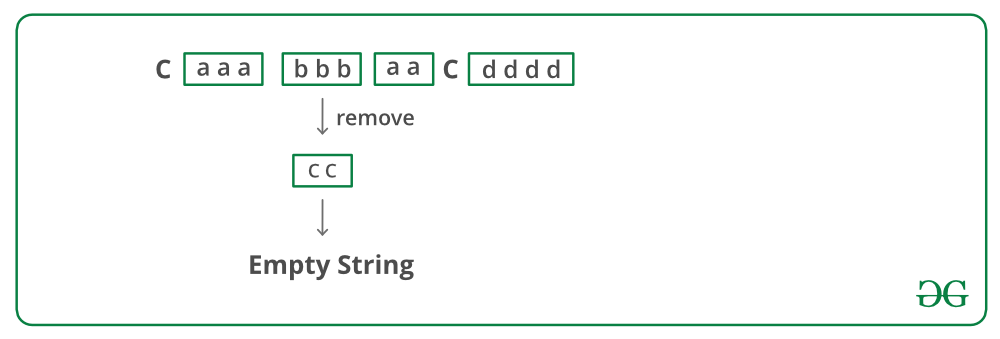# C++ Program To Recursively Remove All Adjacent Duplicates

• Last Updated : 23 Dec, 2021

Given a string, recursively remove adjacent duplicate characters from the string. The output string should not have any adjacent duplicates. See the following examples.

Examples

Input: azxxzy
Output: ay
First “azxxzy” is reduced to “azzy”.
The string “azzy” contains duplicates,
so it is further reduced to “ay”.

Input: geeksforgeeg
Output: gksfor
First “geeksforgeeg” is reduced to
“gksforgg”. The string “gksforgg”
contains duplicates, so it is further
reduced to “gksfor”.

Input: caaabbbaacdddd
Output: Empty String

Input: acaaabbbacdddd
Output: acac

The following approach can be followed to remove duplicates in O(N) time:

• Start from the leftmost character and remove duplicates at left corner if there are any.
• The first character must be different from its adjacent now. Recur for string of length n-1 (string without first character).
• Let the string obtained after reducing right substring of length n-1 be rem_str. There are three possible cases
1. If first character of rem_str matches with the first character of original string, remove the first character from rem_str.
2. If remaining string becomes empty and last removed character is same as first character of original string. Return empty string.
3. Else, append the first character of the original string at the beginning of rem_str.
• Return rem_str.

Below image is a dry run of the above approach:Below is the implementation of the above approach:

## C++

 `// C/C++ program to remove all ``// adjacent duplicates from a string``#include ``#include `` ` `using` `namespace` `std;`` ` `// Recursively removes adjacent ``// duplicates from str and returns``// new string. las_removed is a ``// pointer to last_removed character``char``* removeUtil(``char` `*str, ``                 ``char` `*last_removed)``{    ``    ``// If length of string is 1 or 0``    ``if` `(str == ``''` `|| str == ``''``)``        ``return` `str;`` ` `    ``// Remove leftmost same characters ``    ``// and recur for remaining ``    ``// string``    ``if` `(str == str)``    ``{``        ``*last_removed = str;``        ``while` `(str && str == str)``            ``str++;``        ``str++;``        ``return` `removeUtil(str, last_removed);``    ``}`` ` `    ``// At this point, the first character ``    ``// is definiotely different ``    ``// from its adjacent. Ignore first ``    ``// character and recursively ``    ``// remove characters from remaining string``    ``char``* rem_str = removeUtil(str+1, ``                               ``last_removed);`` ` `    ``// Check if the first character ``    ``// of the rem_string matches with ``    ``// the first character of the ``    ``// original string``    ``if` `(rem_str && rem_str == str)``    ``{``        ``*last_removed = str;``       ` `        ``// Remove first character``        ``return` `(rem_str+1); ``    ``}`` ` `    ``// If remaining string becomes ``    ``// empty and last removed character``    ``// is same as first character of ``    ``// original string. This is needed``    ``// for a string like "acbbcddc"``    ``if` `(rem_str == ``''` `&& ``                 ``*last_removed == str)``        ``return` `rem_str;`` ` `    ``// If the two first characters ``    ``// of str and rem_str don't match, ``    ``// append first character of str ``    ``// before the first character of``    ``// rem_str. ``    ``rem_str--;``    ``rem_str = str;``    ``return` `rem_str;``}`` ` `// Function to remove``char` `*``remove``(``char` `*str)``{``    ``char` `last_removed = ``''``;``    ``return` `removeUtil(str, &last_removed);``}`` ` `// Driver program to test ``// above functions``int` `main()``{``    ``char` `str1[] = ``"geeksforgeeg"``;``    ``cout << ``remove``(str1) << endl;`` ` `    ``char` `str2[] = ``"azxxxzy"``;``    ``cout << ``remove``(str2) << endl;`` ` `    ``char` `str3[] = ``"caaabbbaac"``;``    ``cout << ``remove``(str3) << endl;`` ` `    ``char` `str4[] = ``"gghhg"``;``    ``cout << ``remove``(str4) << endl;`` ` `    ``char` `str5[] = ``"aaaacddddcappp"``;``    ``cout << ``remove``(str5) << endl;`` ` `    ``char` `str6[] = ``"aaaaaaaaaa"``;``    ``cout << ``remove``(str6) << endl;`` ` `    ``char` `str7[] = ``"qpaaaaadaaaaadprq"``;``    ``cout << ``remove``(str7) << endl;`` ` `    ``char` `str8[] = ``"acaaabbbacdddd"``;``    ``cout << ``remove``(str8) << endl;`` ` `    ``char` `str9[] = ``"acbbcddc"``;``    ``cout << ``remove``(str9) << endl;`` ` `    ``return` `0;``}`

Output:

```gksfor
ay
g
a
qrq
acac
a```

Time Complexity: The time complexity of the solution can be written as T(n) = T(n-k) + O(k) where n is length of the input string and k is the number of first characters which are same. Solution of the recurrence is O(n)

Thanks to Prachi Bodke for suggesting this problem and initial solution.

Another Approach:
The idea here is to check whether the String remStr has the repeated character that matches the last char of the parent String. If that is happening then we have to keep removing that character before concatenating string s and string remStr.

Below is the implementation of the above approach:

## C++

 `// C++ Program for above approach``#include ``using` `namespace` `std;`` ` `// Recursively removes adjacent ``// duplicates from str and returns ``// new string. las_removed is a ``// pointer to last_removed character``string removeDuplicates(string s,``                        ``char` `ch)``{``    ``// If length of string is 1 or 0``    ``if` `(s.length() <= 1) ``   ``{``        ``return` `s;``    ``}`` ` `    ``int` `i = 0;``    ``while` `(i < s.length()) ``    ``{``        ``if` `(i + 1 < s.length() && ``            ``s[i] == s[i + 1]) ``        ``{``            ``int` `j = i;``            ``while` `(j + 1 < s.length() && ``                   ``s[j] == s[j + 1]) ``            ``{``                ``j++;``            ``}``            ``char` `lastChar = i > 0 ? s[i - 1] : ch;`` ` `            ``string remStr = removeDuplicates(``                   ``s.substr(j + 1, s.length()), lastChar);`` ` `            ``s = s.substr(0, i);``            ``int` `k = s.length(), l = 0;`` ` `            ``// Recursively remove all the adjacent``            ``// characters formed by removing the``            ``// adjacent characters``            ``while` `(remStr.length() > 0 && ``                   ``s.length() > 0 && ``                   ``remStr == s[s.length() - 1]) ``            ``{``                ``// Have to check whether this is the``                ``// repeated character that matches the``                ``// last char of the parent String``                ``while` `(remStr.length() > 0 && ``                       ``remStr != ch && ``                       ``remStr == s[s.length() - 1]) ``                ``{``                    ``remStr = remStr.substr(1, ``                             ``remStr.length());``                ``}``                ``s = s.substr(0, s.length() - 1);``            ``}``            ``s = s + remStr;``            ``i = j;``        ``}``        ``else` `        ``{``            ``i++;``        ``}``    ``}``    ``return` `s;``}`` ` `// Driver Code``int` `main()``{``    ``string str1 = ``"mississipie"``;``    ``cout << removeDuplicates(str1, ``' '``) << ``            ``endl;`` ` `    ``string str2 = ``"ocvvcolop"``;``    ``cout << removeDuplicates(str2, ``' '``) << ``            ``endl;``}``// This code is contributed by nirajgusain5`

Output:

```mpie
lop```

Time Complexity: O(n)

Please refer complete article on Recursively remove all adjacent duplicates for more details!

My Personal Notes arrow_drop_up